Navigation C API Pages Python bindings Applications

## Pixmap filters

Pixel filters for gp_pixmap.

The pixmap filter is basically a function that operates on pixmap pixels. The result may be stored into a new bitmap or placed to bitmap passed as argument or, in some cases, the filter could be used in place so the result is stored into the same pixmap as the one passed as filter source.

### Common filter API

For convenience, the filters API is unified:

• There are two functions for each filter

• first one takes destination as an argument

• second one allocates the destination and returns pointer to it

• First argument(s) are always source(s)

• Then, in case of second variant, destination

• Other parameters follow

• And the last argument is progress callback

When using allocating version of the filter, pointer to the newly allocated pixmap is returned, or in case of failure NULL is returned.

If malloc() has failed NULL is returned.

If filter has been interrupted by a callback, all allocated memory is freed, and NULL is returned.

When using non-allocating variant of the filter, the destination pixmap must have correct pixel type and the size must be big enough to store the result. The return value from such filter is either zero, in case of success, or non-zero when filter was interrupted by a callback.

For filters that work in-place (which is explicitly said for each filter) the source and the destination could be the same pixmap. Note that this is not expected to work if you do several overlapping sub-pixmaps and pass these as arguments. Filter used in-place may also cause the filter to run single-threaded which is explicit limitation for all but point filters.

```/*
* Filter common API.
*/
int gp_filter_foo(const gp_pixmap *src, gp_pixmap *dst,
foo params ...,
gp_progress_cb *callback);

gp_pixmap *gp_filter_foo_alloc(const gp_pixmap *src,
foo params ...,
gp_progress_cb *callback);```

### Point Filters

Point operations are filters that works with pixels as with independent values (the value of destination pixel depends only on the pixel on the same coordinates in source image). All of these filters works in-place and the result has always the same size as the source.

#### Invert

```#include <gfxprim.h>
/* or */
#include <filters/gp_point.h>

int gp_filter_invert(const gp_pixmap *src, gp_pixmap *dst,
gp_progress_cb *callback);

gp_pixmap *gp_filter_invert_alloc(const gp_pixmap *src,
gp_progress_cb *callback);```

The pixel channel values are counted as `chann_max - val`.

#### Brightness

```#include <gfxprim.h>
/* or */
#include <filters/gp_point.h>

int gp_filter_brightness(const gp_pixmap *src, gp_pixmap *dst,
float p, gp_progress_cb *callback);

gp_pixmap *gp_filter_brightness_alloc(const gp_pixmap *src, float p,
gp_progress_cb *callback);```

The pixel channel values are counted as `val + chann_max * p`.

#### Contrast

```#include <gfxprim.h>
/* or */
#include <filters/gp_point.h>

int gp_filter_contrast(const gp_pixmap *src, gp_pixmap *dst,
float p, gp_progress_cb *callback);

gp_pixmap *gp_filter_contrast_alloc(const gp_pixmap *src, float p,
gp_progress_cb *callback);```

The pixel channel values are counted as `val * p`.

#### BrightnessContrast

```#include <gfxprim.h>
/* or */
#include <filters/gp_point.h>

int gp_filter_brightness_contrast(const gp_pixmap *src, gp_pixmap *dst,
float b, float c,
gp_progress_cb *callback);

gp_pixmap *gp_filter_brightness_contrast_alloc(const gp_pixmap *src,
float b, float c,
gp_progress_cb *callback);```

The pixel channel values are counted as `val * c + chann_max * b`.

Original Image; BrightnessContrast b=-0.2 c=0.8, b=-0.5 c=2, b=0.2 c=0.8, b=0.2 c=1.5

#### Posterize

```#include <gfxprim.h>
/* or */
#include <filters/gp_point.h>

int gp_filter_posterize(const gp_pixmap *src, gp_pixmap *dst,
unsigned int levels, gp_progress_cb *callback);

gp_pixmap *gp_filter_posterize_alloc(const gp_pixmap *src, unsigned int levels,
gp_progress_cb *callback);```

The pixel channel values are quantized into number of levels.

```#include <gp_filter_s.h>
/* or */
#include <filters/gp_gaussian_noise.h>

gp_coord x_src, gp_coord y_src,
gp_size w_src, gp_size h_src,
gp_pixmap *dst,
gp_coord x_dst, gp_coord y_dst,
float sigma, float mu,
gp_progress_cb *callback);

gp_coord x_src, gp_coord y_src,
gp_size w_src, gp_size h_src,
float sigma, float mu,
gp_progress_cb *callback);

static inline int gp_filter_gaussian_noise_add(const gp_pixmap *src,
gp_pixmap *dst,
float sigma, float mu,
gp_progress_cb *callback);

static inline gp_pixmap *
float sigma, float mu,
gp_progress_cb *callback);```

Gaussian additive noise filter adds gaussian distributed noise to an image with a defined sigma and mu. Both sigma and mu weights mapped to [0,1] interval.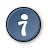See the gaussian noise example.
Original Image; Gaussian Additive Noise s=0.03 m=0, s=0.05 m=0, s=0.05 m=-0.1, s=0.05 m=0.1, s=0.07 m=0.0

### Arithmetic filters

Arithmetic filters do take two pixmaps as an input and combines them into one output pixmap.

The pixel type of both input pixmaps must match.

If size of the input pixmaps differs, minimum is used.

```#include <filters/gp_arithmetic.h>
/* or */
#include <gfxprim.h>

const gp_pixmap *src_b,
gp_pixmap *dst,
gp_progress_cb *callback);

const gp_pixmap *src_b,
gp_progress_cb *callback);```

Produces saturated (clamped) addition of two pixmaps.

```#include <filters/gp_arithmetic.h>
/* or */
#include <gfxprim.h>

int gp_filter_multiply(const gp_pixmap *src_a,
const gp_pixmap *src_b,
gp_pixmap *dst,
gp_progress_cb *callback);

gp_pixmap *gp_filter_multiply_alloc(const gp_pixmap *src_a,
const gp_pixmap *src_b,
gp_progress_cb *callback);```

Produces saturated (clamped) multiplication of two pixmaps.

```#include <filters/GP_Arigthmetic.h>
/* or */
#include <gfxprim.h>

int gp_filter_difference(const gp_pixmap *src_a,
const gp_pixmap *src_b,
gp_pixmap *dst,
gp_progress_cb *callback);

gp_pixmap *gp_filter_difference_alloc(const gp_pixmap *src_a,
const gp_pixmap *src_b,
gp_progress_cb *callback);
```

Produces symmetric difference (i.e. abs(a - b)).

```#include <filters/GP_Arigthmetic.h>
/* or */
#include <gfxprim.h>

int gp_filter_max(const gp_pixmap *src_a,
const gp_pixmap *src_b,
gp_pixmap *dst,
gp_progress_cb *callback);

gp_pixmap *gp_filter_max_alloc(const gp_pixmap *src_a,
const gp_pixmap *src_b,
gp_progress_cb *callback);

int gp_filter_min(const gp_pixmap *src_a,
const gp_pixmap *src_b,
gp_pixmap *dst,
gp_progress_cb *callback);

gp_pixmap *gp_filter_min_alloc(const gp_pixmap *src_a,
const gp_pixmap *src_b,
gp_progress_cb *callback);```

Maximum and minimum filter.

### Rotation and Symmetry filters

```#include <filters/gp_rotate.h>
/* or */
#include <gfxprim.h>

int gp_filter_mirror_h(const gp_pixmap *src, gp_pixmap *dst,
gp_progress_cb *callback);

gp_pixmap *gp_filter_mirror_h_alloc(const gp_pixmap *src,
gp_progress_cb *callback);```

Mirrors pixmap horizontally.

Works in-place.

The destination has to have the same pixel type and the size must be at least as large as source.

Original Image; Mirrored Horizontally
```#include <filters/gp_rotate.h>
/* or */
#include <gfxprim.h>

int gp_filter_mirror_v(const gp_pixmap *src, gp_pixmap *dst,
gp_progress_cb *callback);

gp_pixmap *gp_filter_mirror_v_alloc(const gp_pixmap *src,
gp_progress_cb *callback);```

Mirrors pixmap vertically.

Works in-place.

The destination has to have the same pixel type and the size must be at least as large as source.

Original Image; Mirrored Vertically
```#include <filters/gp_rotate.h>
/* or */
#include <gfxprim.h>

int gp_filter_rotate_90(const gp_pixmap *src, gp_pixmap *dst,
gp_progress_cb *callback);

gp_pixmap *gp_filter_rotate_90_alloc(const gp_pixmap *src,
gp_progress_cb *callback);```

Rotate pixmap by 90 degrees.

Doesn’t work in-place (yet).

The destination has to have the same pixel type and size must be large enough to fit rotated pixmap (i.e. W and H are swapped).

Original Image; Rotated by 90 degrees
```#include <filters/gp_rotate.h>
/* or */
#include <gfxprim.h>

int gp_filter_rotate_180(const gp_pixmap *src, gp_pixmap *dst,
gp_progress_cb *callback);

gp_pixmap *gp_filter_rotate_180_alloc(const gp_pixmap *src,
gp_progress_cb *callback);```

Rotate pixmap by 180 degrees.

Doesn’t work in-place (yet).

The destination has to have the same pixel type and the size must be at least as large as source.

Original Image; Rotated by 180 degrees
```#include <filters/gp_rotate.h>
/* or */
#include <gfxprim.h>

int gp_filter_rotate_270(const gp_pixmap *src, gp_pixmap *dst,
gp_progress_cb *callback);

gp_pixmap *gp_filter_rotate_270_alloc(const gp_pixmap *src,
gp_progress_cb *callback);```

Rotate pixmap by 270 degrees.

Doesn’t work in-place (yet).

The destination has to have the same pixel type and destination size must be large enough to fit rotated pixmap (i.e. W and H are swapped).

Original Image; Rotated by 270 degrees
```#include <filters/gp_rotate.h>
/* or */
#include <gfxprim.h>

typedef enum gp_filter_symmetries {
GP_ROTATE_90,
GP_ROTATE_CW = GP_ROTATE_90,
GP_ROTATE_180,
GP_ROTATE_270,
GP_ROTATE_CCW = GP_ROTATE_270,
GP_MIRROR_H,
GP_MIRROR_V,
} gp_filter_symmetries;

gp_pixmap *gp_filter_symmetry_alloc(const gp_pixmap *src,
gp_filter_symmetries symmetry,
gp_progress_cb *callback);

int gp_filter_symmetry(const gp_pixmap *src, gp_pixmap *dst,
gp_filter_symmetries symmetry,
gp_progress_cb *callback);```

Catch all function for symmetry filters.

### Linear filters

Linear filters family consists of filters based on discrete linear convolution, that means that computed pixel value depends on linear combination of the image pixels.

It’s defined as: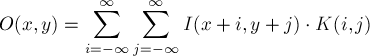The K denotes convolution kernel and in practice, due to computational complexity i and j are bounded in relatively small intervals. For example i and j are in (-1,1) and the kernel size is 3x3.

Note that pixel values outside the image are undefined. The linear convolution in GFXprim simply uses the closest border pixel values for all pixels outside the image.

Particular convolution kernel is called separable if it could be decomposed into two one dimensional kernels (these when combined yields back the original kernel). Such convolution then could be applied as two one dimensional convolutions which is faster operation (especially for big kernels).

#### Bilinear Convolution

Following paragraph describes linear convolution implementation as well as a little of the math background skip it if you just need to use one of the ready-to-use filters.

```#include <filters/gp_linear.h>
/* or */
#include <gfxprim.h>

int gp_filter_linear_convolution_raw(const gp_pixmap *src,
gp_coord x_src, gp_coord y_src,
gp_size w_src, gp_size h_src,
gp_pixmap *dst,
gp_coord x_dst, gp_coord y_dst,
float kernel[], uint32_t kw, uint32_t kh,
float kern_div, gp_progress_cb *callback);```

Internal generic convolution filter, this is a base for all linear convolution filters with non-separable kernel.

The src coordinate and sizes denotes rectangle in the source pixmap that the filter operates on.

The dst coordinates defines offset into the dst pixmap.

The kernel is two-dimensional array of a size kw * kh indexed as kernel[x + y*kw].

The kern_div is a coefficient that is used to divide the resulting values often used to normalize the result.

This filter works in-place.

The pixel value is computed as: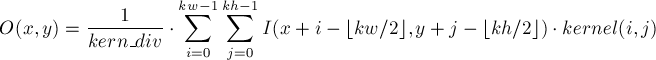Which is the same as: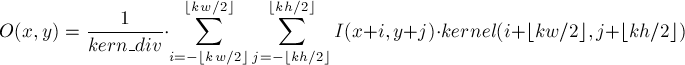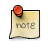The number of kernel rows and columns is expected to be odd number.
```#include <filters/gp_linear.h>
/* or */
#include <gfxprim.h>

int gp_filter_hlinear_convolution_raw(const gp_pixmap *src,
gp_coord x_src, gp_coord y_src,
gp_size w_src, gp_size h_src,
gp_pixmap *dst,
gp_coord x_dst, gp_coord y_dst,
float kernel[], uint32_t kw, float kern_div,
gp_progress_cb *callback);

int gp_filter_vlinear_convolution_raw(const gp_pixmap *src,
gp_coord x_src, gp_coord y_src,
gp_size w_src, gp_size h_src,
gp_pixmap *dst,
gp_coord x_dst, gp_coord y_dst,
float kernel[], uint32_t kh, float kern_div,
gp_progress_cb *callback);

int gp_filter_vhlinear_convolution_raw(const gp_pixmap *src,
gp_coord x_src, gp_coord y_src,
gp_size w_src, gp_size h_src,
gp_pixmap *dst,
gp_coord x_dst, gp_coord y_dst,
float hkernel[], uint32_t kw, float hkern_div,
float vkernel[], uint32_t kh, float vkern_div,
gp_progress_cb *callback);

void gp_filter_kernel_print_raw(float kernel[], int kw, int kh, float kern_div);```

Internal special functions for one dimensional vertical and horizontal convolution these two functions are base for all separable convolution filters.

The src coordinate and sizes denotes rectangle in the source pixmap that the filter operates on.

The dst coordinates are offset into the dst.

The kernel is one-dimensional array of floats of size kw or kh.

The kern_div is a coefficient that is used to divide the resulting values.

The last function does both vertical and horizontal convolution and takes care of correct progress callback.

These filters work in-place.

The pixel value is computed as: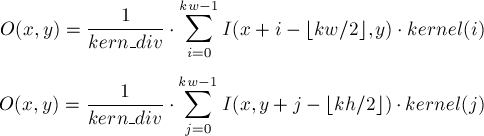Which is the same as: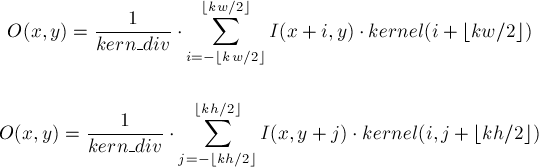The number of kernel rows and columns is expected to be odd number.The linear convolutions are internally implemented using integer arithmetics, which works fine, but you need to take a care not to overflow 32bit signed integer. If the pixel channel size is 8bit long and 10bits are used for the fixed point part of the number the rest must fit into about 10 bits to be safe.
```#include <filters/gp_convolution.h>
/* or */
#include <gfxprim.h>

typedef struct gp_filter_kernel_2d {
unsigned int w;
unsigned int h;
float div;
float *kernel;
} gp_filter_kernel_2d;

int gp_filter_convolution_ex(const gp_pixmap *src,
gp_coord x_src, gp_coord y_src,
gp_size w_src, gp_coord h_src,
gp_pixmap *dst,
gp_coord x_dst, gp_coord y_dst,
const gp_filter_Kernel2D *kernel,
gp_progress_cb *callback);

gp_pixmap *gp_filter_convolution_ex_alloc(const gp_pixmap *src,
gp_coord x_src, gp_coord y_src,
gp_size w_src, gp_size h_src,
const gp_filter_Kernel2D *kernel,
gp_progress_cb *callback);

int gp_filter_convolution(const gp_pixmap *src, gp_pixmap *dst,
const gp_filter_Kernel2D *kernel,
gp_progress_cb *callback);

gp_pixmap *gp_filter_convolution_alloc(const gp_pixmap *src,
const gp_filter_Kernel2D *kernel,
gp_progress_cb *callback);

void gp_filter_kernel_2d_print(const gp_filter_kernel_2d *kernel);```

Linear convolution filters, you should preferably use this API over the _Raw variants.

The _ex variants takes a rectangle on which the filter should operate as well as offset into the destination. The destination must be large enough so that starting with offset there is at least w_dst and h_dst pixels.

The kernel is a pointer to a structure initialized with the kernel size, divider and array of kernel values.

The last function prints convolution kernel in human-readable format into the stdout.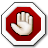If filter is executed in-place the work cannot be distributed between threads (as some of the threads will overwrite values read by other threads). In this case convolution filters runs in one thread regardless of if threads are eanbled or not.
```#include <gfxprim.h>

/*
* _example box smoothing filter.
*/
static void box_smoothing(gp_pixmap *img)
{
float box_filter[] = {
1, 1, 1,
1, 1, 1,
1, 1, 1,
};

gp_filter_kernel_2d box_kernel = {
.w = 3,
.h = 3,
.div = 9,
.kernel = box_filter,
};

gp_filter_convolution(img, img, &box_kernel, NULL);
}```

_example function that implements simple in-place smoothing filter.

#### Laplace Filter

```#include <gp_filter_s.h>
/* or */
#include <gfxprim.h>

int gp_filter_laplace(const gp_pixmap *src, gp_pixmap *dst,
gp_progress_cb *callback);

gp_pixmap *gp_filter_laplace_alloc(const gp_pixmap *src,
gp_progress_cb *callback);```

Discrete Laplace filter that produces a second derivative of the original image.

The convolution kernel is defined as: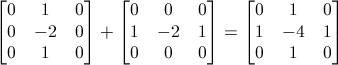This filter is not separable but could be written as a sum of two one dimensional filters as the kernel definition suggests.

#### Laplacian Edge Sharpening

```#include <gp_filter_s.h>
/* or */
#include <gfxprim.h>

int gp_filter_edge_sharpening(const gp_pixmap *src, gp_pixmap *dst,
float w, gp_progress_cb *callback);

gp_pixmap *gp_filter_edge_sharpening_alloc(const gp_pixmap *src, float w,
gp_progress_cb *callback);```

Laplace based edge sharpening filter, subtracts weighted second derivative from the original image.

The w paramerter is multiplicative weight applied on the second derivative. Reasonable results are when the parameter is between 0.1 and 1.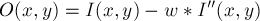#### Gaussian Blur

```#include <filters/gp_blur.h>
/* or */
#include <gfxprim.h>

int gp_filter_gaussian_blur_ex(const gp_pixmap *src,
gp_coord x_src, gp_coord y_src,
gp_size w_src, gp_size h_src,
gp_pixmap *dst,
gp_coord x_dst, gp_coord y_dst,
float x_sigma, float y_sigma,
gp_progress_cb *callback);

gp_pixmap *gp_filter_gaussian_blur_ex_alloc(const gp_pixmap *src,
gp_coord x_src, gp_coord y_src,
gp_size w_src, gp_size h_src,
float x_sigma, float y_sigma,
gp_progress_cb *callback);

int gp_filter_gaussian_blur(const gp_pixmap *src, gp_pixmap *dst,
float x_sigma, float y_sigma,
gp_progress_cb *callback)

gp_pixmap *gp_filter_gaussian_blur_alloc(const gp_pixmap *src,
float x_sigma, float y_sigma,
gp_progress_cb *callback)```

Gaussian blur (low pass) filters implemented as bilinear separable convolution.

The sigma denotes amount of the blur (the radius is computed accordingly automatically).

The sigma values can be set for vertical and horizontal direction independently which may be useful when Gaussian blur is used as a low pass filter before image is resampled non proportionally.

Original Image; Gaussian Blur xsig=2 ysig=2, xsig=0 ysig=4, xsig=4 ysig=0, xsig=4 ysig=4, xsig=10 ysig=10

### Interpolation filters

Filters to resize image.

#### Nearest Neighbour Interpolation

Fast, but produces "pixelated" images. May however work better for images with sharp edges mostly consisting of big one color regions (it doesn’t blur the result on upscaling).

Also is commonly used to show preview before you resample the image correctly.

#### Bilinear Interpolation

Bilinear is faster than bicubic interpolation and produces quite good results especially the low pass variant doesn’t need additional filter on down-sampling.

#### Bicubic Interpolation

Works well as is on image upscaling. To get decent result on downscaling low-pass filter (Gaussian blur) must be used on original image before actual downscaling. To do this reasonably fast we could cheat a little: first resize big images a little without the low-pass filter, then apply low-pass filter and finally downscale it to desired size.

### Dithering

Dithering filters are filters for better loosy (source pixel type has more bits to represent color or grayscale value) pixel type conversions.

### Median

```#include <filters/gp_median.h>
/* or */
#include <gfxprim.h>

int gp_filter_median_ex(const gp_pixmap *src,
gp_coord x_src, gp_coord y_src,
gp_size w_src, gp_size h_src,
gp_pixmap *dst,
gp_coord x_dst, gp_coord y_dst,
int xmed, int ymed,
gp_progress_cb *callback);

gp_pixmap *gp_filter_median_ex_alloc(const gp_pixmap *src,
gp_coord x_src, gp_coord y_src,
gp_size w_src, gp_size h_src,
int xmed, int ymed,
gp_progress_cb *callback);

int gp_filter_median(const gp_pixmap *src,
gp_pixmap *dst,
int xmed, int ymed,
gp_progress_cb *callback);

gp_pixmap *gp_filter_median_alloc(const gp_pixmap *src,
int xmed, int ymed,
gp_progress_cb *callback);```

Constant time median filter (the computational complexity is independent of radius size).

The xmed and ymed are radius values for x and y. The algorithm uses xmed respectively ymed pixel neighbors from each side so the result is median of rectangle of 2 * xmed + 1 x 2 * ymed + 1 pixels.

Original Image; Median xr=3 yr=3, xr=5 yr=5, xr=7 yr=7, xr=9 yr=9, xr=12 yr=12